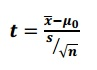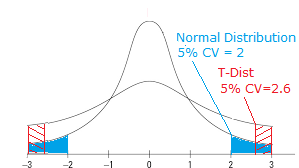# Degrees of Freedom: What are they?

Share on

Degrees of freedom are used in hypothesis testing.

Watch the video for an overview of degrees of freedom and why we subtract 1:

## What are Degrees of Freedom?

Degrees of freedom of an estimate is the number of independent pieces of information that went into calculating the estimate. It’s not quite the same as the number of items in the sample. In order to get the df for the estimate, you have to subtract 1 from the number of items. Let’s say you were finding the mean weight loss for a low-carb diet. You could use 4 people, giving 3 degrees of freedom (4 – 1 = 3), or you could use one hundred people with df = 99.

In math terms (where “n” is the number of items in your set):

Degrees of Freedom = n – 1

Why do we subtract 1 from the number of items?

Another way to look at degrees of freedom is that they are the number of values that are free to vary in a data set. What does “free to vary” mean? Here’s an example using the mean (average):
Q. Pick a set of numbers that have a mean (average) of 10.
A. Some sets of numbers you might pick: 9, 10, 11 or 8, 10, 12 or 5, 10, 15.
Once you have chosen the first two numbers in the set, the third is fixed. In other words, you can’t choose the third item in the set. The only numbers that are free to vary are the first two. You can pick 9 + 10 or 5 + 15, but once you’ve made that decision you must choose a particular number that will give you the mean you are looking for. So degrees of freedom for a set of three numbers is TWO.

For example: if you wanted to find a confidence interval for a sample, degrees of freedom is n – 1. “N’ can also be the number of classes or categories. See: Critical chi-square value for an example.

## Degrees of Freedom: Two Samples

If you have two samples and want to find a parameter, like the mean, you have two “n”s to consider (sample 1 and sample 2). Degrees of freedom in that case is:

Degrees of Freedom (Two Samples): (N1 + N2) – 2.

## Degrees of Freedom in ANOVA

Degrees of freedom becomes a little more complicated in ANOVA tests. Instead of a simple parameter (like finding a mean), ANOVA tests involve comparing known means in sets of data. For example, in a one-way ANOVA you are comparing two means in two cells. The grand mean (the average of the averages) would be:
Mean 1 + mean 2 = grand mean.
What if you chose mean 1 and you knew the grand mean? You wouldn’t have a choice about Mean2, so your degrees of freedom for a two-group ANOVA is 1.

Two Group ANOVA df1 = n – 1

For a three-group ANOVA, you can vary two means so degrees of freedom is 2.

It’s actually a little more complicated because there are two degrees of freedom in ANOVA: df1 and df2. The explanation above is for df1. Df2 in ANOVA is the total number of observations in all cells – degrees of freedoms lost because the cell means are set.

Two Group ANOVA df2 = n – k

The “k” in that formula is the number of cell means or groups/conditions.
For example, let’s say you had 200 observations and four cell means. Degrees of freedom in this case would be: Df2 = 200 – 4 = 196.

## Why Do Critical Values Decrease While DF Increase?

Thanks to Mohammed Gezmu for this question.

Let’s take a look at the t-score formula in a hypothesis test:When n increases, the t-score goes up. This is because of the square root in the denominator: as it gets larger, the fraction s/√n gets smaller and the t-score (the result of another fraction) gets bigger. As the degrees of freedom are defined above as n-1, you would think that the t-critical value should get bigger too, but they don’t: they get smaller. This seems counter-intuitive.

However, think about what a t-test is actually for. You’re using the t-test because you don’t know the standard deviation of your population and therefore you don’t know the shape of your graph. It could have short, fat tails. It could have long skinny tails. You just have no idea. The degrees of freedom affect the shape of the graph in the t-distribution; as the df get larger, the area in the tails of the distribution get smaller. As df approaches infinity, the t-distribution will look like a normal distribution. When this happens, you can be certain of your standard deviation (which is 1 on a normal distribution).

Let’s say you took repeated sample weights from four people, drawn from a population with an unknown standard deviation. You measure their weights, calculate the mean difference between the sample pairs and repeat the process over and over. The tiny sample size of 4 will result a t-distribution with fat tails. The fat tails tell you that you’re more likely to have extreme values in your sample. You test your hypothesis at an alpha level of 5%, which cuts off the last 5% of your distribution. The graph below shows the t-distribution with a 5% cut off. This gives a critical value of 2.6. (Note: I’m using a hypothetical t-distribution here as an example--the CV is not exact).Now look at the normal distribution. We have less chance of extreme values with the normal distribution. Our 5% alpha level cuts off at a CV of 2.

Back to the original question “Why Do Critical Values Decrease While DF Increases?” Here’s the short answer:

Degrees of freedom are related to sample size (n-1). If the df increases, it also stands that the sample size is increasing; the graph of the t-distribution will have skinnier tails, pushing the critical value towards the mean.

Reference:
Gerard Dallal. The Little Handbook of Statistical Practice. Retrieved December 26 2015 from here.
Alistair W Kerr, Howard K Hall, Stephen A Kozub. (2002). Doing Statistics with SPSS. Sage Publications. p.68. Available here.
Levine, D. (2014). Even You Can Learn Statistics and Analytics: An Easy to Understand Guide to Statistics and Analytics 3rd Edition. Pearson FT Press

CITE THIS AS:
Stephanie Glen. "Degrees of Freedom: What are they?" From StatisticsHowTo.com: Elementary Statistics for the rest of us! https://www.statisticshowto.com/probability-and-statistics/hypothesis-testing/degrees-of-freedom/
---------------------------------------------------------------------------Need help with a homework or test question? With Chegg Study, you can get step-by-step solutions to your questions from an expert in the field. Your first 30 minutes with a Chegg tutor is free!